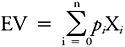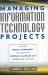# Quantifying Risks - The Fourth Step

## Quantifying Risks—The Fourth Step

Assigning a percentage value for risk probability creates opportunities for risk comparisons that wouldn't otherwise exist. The most difficult task throughout a risk analysis is estimating the probability of occurrence of the event with any degree of accuracy. One can argue that assigning a percentage of probability is very subjective and, therefore, inaccurate. However, it must be remembered that risks do not have to be measured in absolute terms to be of value; they can be measured relative to each other.

The most commonly used tool for determining the relative value of project risks is expected value. Expected value (EV) is a technique useful in assessing different technical approaches or making trade-off analyses. The concept is based on the probability of different outcomes occurring and explicitly considers the risk of the different approaches. That is, if there is a chance that one of several outcomes for a given scenario can occur, depending on the risks involved for each of the different conditions, then the most likely outcome over time can be determined. This most likely result is known as the expected value.

Expected value is based on the notion that each result is mutually exclusive. That is, the outcome is a random occurrence. A probability can be assigned to that outcome. Mathematically, expected value can be defined in this fashion. Suppose a random variable X, defined if the set of its possible values is given and if the probability of each value's appearance is also given, possesses n values. Then, these possible values (p) can be represented as X1, X2,...Xn.

If p1,, p2,... pn are the probabilities associated with each X, then the expected value of X can be represented by the equation:The interpretation of this equation is that the expected value of an event is the sum of all the possible values of the variable multiplied by the probability of each of those values occurring. Expected value is generally used in conjunction with decision tree analyses, but can be used alone. The key point about expected value is that it is most effective when it is used to compare two or more potential outcomes, which helps eliminate some of the subjectivity in a risk analysis. An example will demonstrate how expected value is used.

### Example

Your company runs a bus service in a midsize city. Currently, the company is using two bus models, both of which have exhibited frame defects over the past five years. The frame repairs cost an average of \$500 per failure, but there has been only one failure per bus during a given year. The following table summarizes the data that have been collected on the frame failures.

Year

Model A (250 buses) Frame Repairs

Model B (175 buses) Frame Repairs

1

10

8

2

21

12

3

34

16

4

54

25

5

63

45

The company is considering replacing the current fleet with 500 new buses using either Model A or B. If Model A's original cost was \$61,000 and Model B's cost was \$64,000, with salvage values of \$8,000 and \$12,000, respectively, what would be the expected cost of each model? Assume that routine yearly repair costs for each model are the same. Using the five-year study data, decide which bus model is the best for the company based on expected cost as the decision criterion. Assume a 10 percent rate of return to calculate the net present value.

#### Solution

The first step in determining the expected cost for each bus is to figure the probability of failure occurrence. In this case, probability can be calculated for each year by dividing the number of failures per year by the total number of failures in each model. For example, in year one, Model A had ten frame failures requiring repair. The probability of a recurrence in year one for the new fleet of Model A buses will be 10/250 = .04, or 4 percent (number of failures divided by fleet size). For Model B, it will be 8/175 = .05, or 5 percent. The table below provides the probability of occurrence for each year and each model.

Year

Model A

Model B

Failures

Probability

Failures

Probability

1

10

0.04

8

0.05

2

21

0.08

12

0.07

3

34

0.14

16

0.09

4

54

0.22

25

0.14

5

63

0.25

34

0.19

The expected value of the repairs during year one for the new fleet of 500 buses can now be calculated by multiplying the probability of occurrence by the number of buses and by the average cost of each repair. Hence, for Model A, year one:

EV = .04 500 \$500 = \$10,000

The following table summarizes the expected value for each year and each model.

Year

Model A

Model B

Expected Value

(probability cost no. buses)

Expected Value

(probability cost no. buses)

1

10,000.00

12,500.00

2

20,000.00

17,500.00

3

35,000.00

22,500.00

4

55,000.00

35,000.00

5

62,500.00

47,500.00

Now determining the original cost and salvage value, we can construct an incremental table so that we can determine which of the two bus models represents the best value to the company. The original cost for Model A is \$61,000, and for Model B it is \$64,000. The salvage value for Model A is \$8,000 per bus, and for Model B it is \$12,000 per bus. So for 500 buses:

Model A

Model B

Original Cost

30,500,000.00

32,000,000.00

Salvage Value

4,000,000.00

6,000,000.00

The differential costs and benefits between the two models are:

Year

Model A

Model B

Increment (B - A)

Original Cost

0

- 30,500,000

- 32,000,000

- 1,500,000

1

- 10,000

- 12,500

- 2,500

2

- 20,000

- 17,500

2,500

3

- 35,000

- 22,500

12,500

4

- 55,000

- 35,000

20,000

5

- 62,500

- 47,500

15,000

Salvage Value

5

+ 4,000,000

+ 6,000,000

2,000,000

Calculating the net present value (NPV) will complete this analysis. But we must discuss present value (PV) first.

Present value is a financial calculation that takes into account the time value of money. That is, it is a way to estimate the future value of an investment or benefit as it is discounted over time. Mathematically, present value is determined by:

PV = FV/(1 + i)t

Where:

 PV = present value of money FV = future value of money i = interest rate (often this is the internal rate of return or the cost of capital) t = time period n = number of time periods ∑ = sum of PV of all the investments or benefits

NPV is defined as the difference between the present value of the benefits and the present value of the investments or costs. Mathematically, NPV is:

NPV = PV(Benefits) - PV(Investments)

A positive NPV is considered good since the benefits out-weigh the investments; a negative result indicates that the venture (or project) is not a good investment.

Using the incremental differences in the analysis of the two buses, the NPV can be found by:

 NPV = - 1,500,000/(1 + .10)0 - 2,500/(1 + .10)1 + 2,500/(1 + .10)2 + 12,500/(1 + .10)3 + 20,000/(1 + .10)4 + 15,000/(1 + .10)5 + 2,000,000/(1 + .10)5 and NPV = - 1,500,000 - 2272.73 + 2,066.12 + 9,391.44 + 13,660.27 + 9,313.82 + 1,241.84 or NPV = - 1,466,599.24

Since the NPV is negative and the increment table was developed so that Model A is subtracted from Model B, that means that Model A has the largest benefit. Hence, the analysis indicates that Model A will be the most cost-effective choice of the two buses.

Notice that had NPV not been used to take into account the time value of money, that is, if a straight comparison between the costs and benefits of the two buses were done, then it would erroneously appear that Model B is the best value.Managing Information Technology Projects: Applying Project Management Strategies to Software, Hardware, and Integration Initiatives
ISBN: 0814408117
EAN: 2147483647
Year: 2003
Pages: 129
Authors: James Taylor

Similar book on Amazon

flylib.com © 2008-2017.
If you may any questions please contact us: flylib@qtcs.net